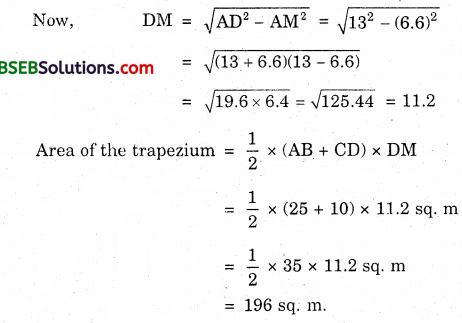# Bihar Board Class 9th Maths Solutions Chapter 12 Heron’s Formula Ex 12.2

Bihar Board Class 9th Maths Solutions Chapter 12 Heron’s Formula Ex 12.2 Textbook Questions and Answers.

## BSEB Bihar Board Class 9th Maths Solutions Chapter 12 Heron’s Formula Ex 12.2Question 1.
A park, in the shape of a quadrilateral ABCD, has ∠C = 90°, AB = 9 m, BC = 12 m, CD = 5 m and AD = 8 m. How much area does it occupy?
Solution: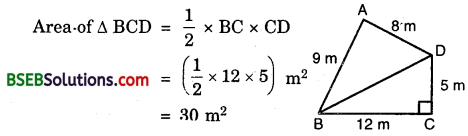Using Pythagoras theorem, we have BD² = BC² + BD²
⇒ BD² = 12² + 5²
⇒ BD² = 144 + 25
⇒ BD = $$\sqrt{169}$$ = 13 m
For : ∆ ABD :
Let a = 13 m, b = 8 m and c = 9 m
s = $$\frac { 1 }{ 2 }$$(a + b + c)
= $$\frac { 1 }{ 2 }$$(13 + 8 + 9) m
= $$\frac { 1 }{ 2 }$$ x 30 m
= 15 m
s – a = (15 – 13) m = 2 m
s – b = (15 – 8) m = 7 m
s – c = (15 – 9) m = 6 m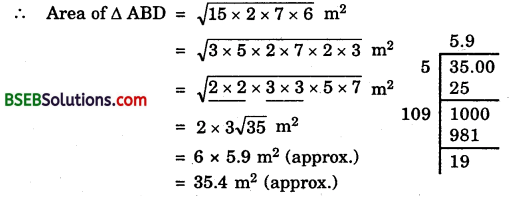∴ Required area = ∆ ABD + ∆ BCD
= 35.4 m² + 30 m²
= 65.4 m²Question 2.
Find the area of a quadrilateral ABCD in which AB = 3 cm, BC = 4 cm, CD = 4 cm, DA = 5 cm and AC = 5 cm.
Solution:
Since AC² = AB² + BC²(as 5² = 3² + 4² i.e., 25 = 9 + 16)
∴ ∠ABC = 90°
Area of rt. ∠d ∆ ABC = $$\frac { 1 }{ 2 }$$ x AB x BC
= ($$\frac { 1 }{ 2 }$$ x 3 x 4) cm² = 6 cm²
For ∆ ACD :
Let a = 5 cm, 6 = 4 cm and c = 5 cm. Then,
s = $$\frac { 1 }{ 2 }$$(a + b + c)
= $$\frac { 1 }{ 2 }$$(5 + 4 + 5) cm
= $$\frac { 1 }{ 2 }$$ x 14 cm
= 7 cm
Now, s – a = (7 – 5) cm = 2 cm
s – b = (7 – 4) cm = 3 cm
s – c = (7 – 5) cm = 2 cmArea of quadrilateral ABCD = Area of ∆ ABC + Area of ∆ ACD = (6 + 9.2) cm² = 15.2 cm² approx.Question 3.
Radha made a picture of an aeroplane with coloured paper as shown in figure. Find the total area of the paper used.
Solution:
Paper used is of triangular shape. Let a = 15 m, b = 11 m and c = 6 m.Now, s = $$\frac { 1 }{ 2 }$$(a + b + c)
= $$\frac { 1 }{ 2 }$$(15 + 11 + 6)m
= $$\frac { 1 }{ 2 }$$ x 32 m = 16 m
= $$\frac { 1 }{ 2 }$$ x 32 m = 16 m
s – a = (16 – 15) m = 1 m
s – b = (16 – 11) m = 5 m
and,
s – c = (16 – 6) m = 10 m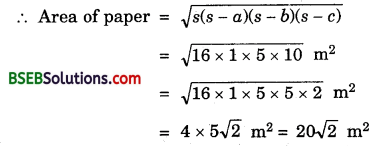Question 4.
A triangle and a parallelogram iiave the same base and the same area. If the sides of the triangle are 26 cm, 28 cm and 30 cm, and the parallelogram stands on the base 28 cm, find the height of the parallelogram.
Solution:
For the triangle :
Let its sides be a, b and c such that a = 26 cm, b = 28 cm and c = 30. Then,
s = $$\frac { 1 }{ 2 }$$(26 + 28 + 30) cm
= $$\frac { 1 }{ 2 }$$ x 84 cm = 42 cm
Now, s – a = (42 – 26) cm = 16 cm
s – b = (42 – 28) cm = 14 cm
and s – c = (42 – 30) cm = 12 cm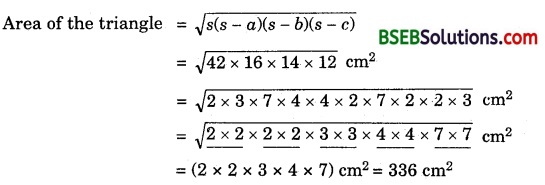For the parallelogram :
Area = base x heightQuestion 5.
A rhombus shaped field has green grass for 18 cows to graze. If each side of the rhombus is 30 m and its longer diagonal is 48 m, how much area of grass field will each cow be getting?
Solution:
We know that the diagonals of the rhombus bisect each other at right angles. Using Pythagoras theorem, we haveArea of one ∆ AOD = ($$\frac { 1 }{ 2 }$$ x 24 x 18) m²
= 216 m²
∴ Area of rhombus = 4 x ∆ AOD
= (4 x 216) m²
= 864 m²
Grass area for 18 cows = 864 m²
Grass area for 1 cows = ($$\frac { 864 }{ 18 }$$) m² = 48 m²Question 6.
An umbrella is made by stitching 10 triangular pieces of cloth of two different colours (See figure), each piece measuring 20 cm, 50 cm and 50 cm. How much cloth of each colour is required for the umbrella?Solution:
In one triangular piece, let a = 20 cm, b = 50 cm and c = 50 cm.
Now, s = $$\frac { 1 }{ 2 }$$(a + b + c) = $$\frac { 1 }{ 2 }$$ (20 + 50 + 50) cm
= $$\frac { 1 }{ 2 }$$ x 120 cm = 60 cm
s – a = (60 – 20) cm = 40 cm
s – b = (60 – 50) cm = 10 cm
and, s – c = (60 – 50) cm = 10 cm
∴ Area of one triangular piece
= $$\sqrt{s(s-a)(s-b)(s-c)}$$
= $$\sqrt{60 \times 40 \times 10 \times 10}$$ cm² = 200$$\sqrt{6}$$ cm²
∴ Area of 5 red triangles = 1000$$\sqrt{6}$$cm²
and, area of 5 green triangles = 1000$$\sqrt{6}$$ cm²Question 7.
A kite in the shape of a square with a diagonal 32 cm and an isosceles triangle of base 8 cm and sides 6 cm each is to be made of three different shades as shown in figure. JBEow much paper of each shade has been used in it?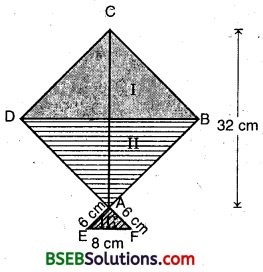Solution:
ABCD is a square such that AC = BC = 32 cm and AEF is an isosceles triangle in which AE = AF = 6 cm
For the area of shades I and II Clearly, from the figure
Area of shade I = Area of shade B = Area of Δ CDB
= $$\frac { 1 }{ 2 }$$ x DB x CM
= $$\frac { 1 }{ 2 }$$ x 32 x 16 sq cm
= 256 sq cm
For the area of shade III (i.e., A AEF)EL = LF = $$\frac { 1 }{ 2 }$$ EM
= $$\frac { 1 }{ 2 }$$ x 8 = 4 cm
and AE = 6 cm (given)
∴ AL = $$\sqrt{\mathrm{AE}^{2}-\mathrm{EL}^{2}}$$
= $$\sqrt{36-16}$$ = $$\sqrt{20}$$ = 2$$\sqrt{5}$$ cm
Area of shade III = $$\frac { 1 }{ 2 }$$ x EF x AL
= $$\frac { 1 }{ 2 }$$ x x 2$$\sqrt{5}$$ sq cm
= 8$$\sqrt{5}$$ sq cm
= 8 x 2.24 sq cm (approx.)
= 17.92 sq cm (approx.)Question 8.
A floral design on a floor is made up of 16 tiles which are triangular, the sides of the triangle being 9 cm, 28 cm and 35 cm (See figure). Find the cost of polishing the tiles at the rate of 50 p per cm².Solution:
For one triangular tile :
Let a = 9 cm, b = 28 cm and c = 35 cm
s = $$\frac { 1 }{ 2 }$$ (a + b + c) = $$\frac { 1 }{ 2 }$$(9 + 28+ 35) cm
= $$\frac { 1 }{ 2 }$$ x 72 cm = 36 cm
s – a = (36 – 9) cm = 27 cm
s – b = (36 – 28) cm = 8 cm
s – c = (36 – 35) cm = 1 cm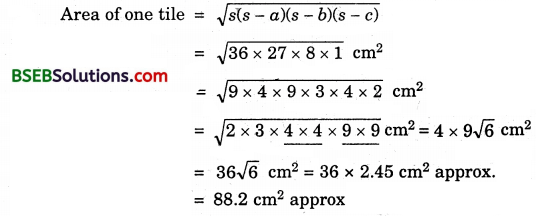∴ Area of 16 such tiles = (16 x 88.2) cm²
= 1411.2 cm² approx.
Cost of polishing @ 50 P per cm² = Rs (1411.2 x $$\frac { 50 }{ 100 }$$)
= Rs 705.60 approx.Question 9.
A field is in the shape of a trapezium whose parallel sides are 25 m and 10 m. The non-parallel sides are 14 m and 13 m. Find the area of the field.
Solution:
Various lengths are as marked in the figure.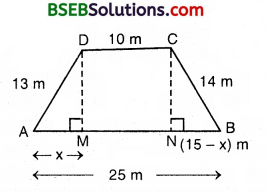Clearly, DM = CN 13 m
⇒ DM² = CN²
⇒ (13)² – x² = 14² – (15 – x)²
⇒ 169 – x² = 196 – (225 – 30x + x²)
⇒ 30x = 169 – 196 + 225 = 198 198
⇒ x = $$\frac { 198 }{ 30 }$$ = 6.6